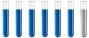## Question on structure of atom

Chemistry and homework help forum.

Organic Chemistry, Analytical Chemistry, Biochemistry, Physical Chemistry, Computational Chemistry, Theoretical Chemistry, High School Chemistry, Colledge Chemistry and University Chemistry Forum.

Share your chemistry ideas, discuss chemical problems, ask for help with scientific chemistry questions, inspire others by your chemistry vision!

Please feel free to start a scientific chemistry discussion here!

Discuss chemistry homework problems with experts!

Ask for help with chemical questions and help others with your chemistry knowledge!

Moderators: expert, ChenBeier, Xen

Dhamnekar Winod
Sr. Staff MemberPosts: 202
Joined: Sat Nov 21, 2020 10:14 am
Location: Mumbai[Bombay],Maharashtra State,India

### Question on structure of atom

An x-ray photoelectron spectroscopy experiment with an unidentified element, X, displays an emission spectrum with four distinct kinetic energies:
5.9 × 10⁻¹⁷ J,2.53 × 10⁻¹⁸ , 2.59 × 10⁻²⁰ J, 2.67 × 10⁻²⁰ (Assume the incident light has sufficient energy to eject any electron in the atom.)

(a)Name all of the possible ground state atoms that could yield this spectrum.

(b)Calculate the binding energy of an electron in the 2p orbital of element X if the x-rays used for the spectroscopy experiment had an energy of $$2.68×10^{−16} J$$

(c)Consider both the filled and unfilled orbitals of element X. Determine the number of: (1) total nodes in a 4d orbital (2)angular nodes in the $$2p_y$$ orbital (3)degenerate 5p orbitals

Energy of photon= work function (ϕ) + KE ⇒ ϕ= Energy of photon-KE ⇒ ϕ= 2.68 × 10⁻¹⁶ - 0.0253 × 10⁻¹⁶ =$$2.6\underline{5}47$$ × 10⁻¹⁶ J , Hence, BE= ϕ= -2.65 × 10⁻¹⁶ J